# Design and Analysis of Hairpin Micro-Strip Line Bandpass Filter

This example shows how to design and analyze Hairpin filter in RF PCB Toolbox™.

Most wireless applications require high quality, low cost, and compact sized RF or microwave filters. Planar filters can be used to fulfill this purpose. Among planar filters hairpin filters are available in reduced size as compared to parallel coupled line structures. These hairpin filters may conceptually be obtained by folding the resonators of parallel-coupled half-wavelength resonator filters. The Hairpin filters are widely used as bandpass filters in transmitters and receivers at various frequencies.

### Create Variables

```N = 3; Ripple = 0.1; BandWidth = 20; Z0 = 50; f = linspace(1.5e9,2.5e9,51); EpsilonR = 6.15; Height = 1.27e-3;```

### Tapped-Input Hairpin Filter

Use the `filterHairpin` object to create the hairpin filter and visualize it.

```filter = filterHairpin; figure; show(filter); view(-37,30);```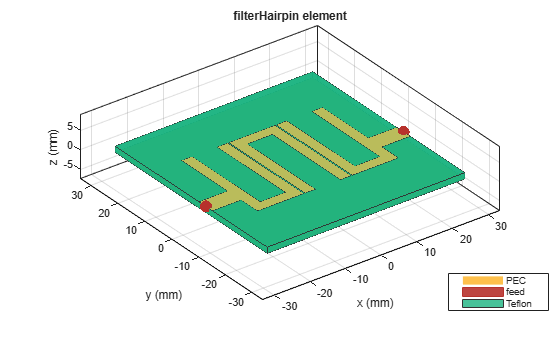Use the `design` function to design a 3rd order hairpin filter with a `RippleFactor` of 0.1 dB and 20% fractional bandwidth and visualize it.

```filter.FilterOrder = N; filter.Height = Height; filter.Substrate.EpsilonR = EpsilonR; filter = design(filter,2e9,'FBW',BandWidth,'RippleFactor',Ripple); figure; show(filter); view(-37,35);```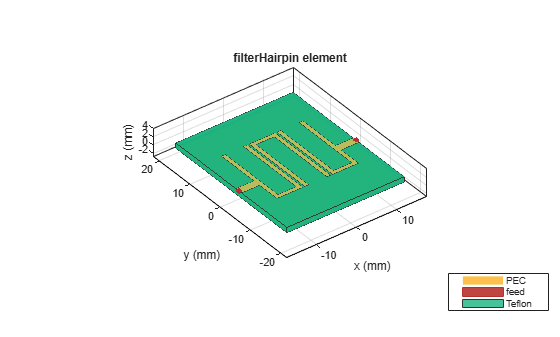Use the `sparameters` function to calculate the s-parameters for the hairpin filter and plot it using `rfplot` function.

```spar = sparameters(filter,f); figure; rfplot(spar);```The result shows that the filter resonates close to the design frequency of 2 GHz. The design function uses analytical equations and the spacing between the resonators is generally calculated using the practical approach. Hence the design function sets the spacing to 0.5 mm between all the resonators. To get the accurate results, the spacing between the resonators needs to be adjusted. Increase the bandwidth of the filter by reducing the spacing between the resonators.

Set the `Spacing` between the resonators to 0.05 mm.

`filter.Spacing = [0.05e-3 0.05e-3];`

Use the `sparameters` function to calculate the s-parameters for the hairpin filter and plot it using `rfplot` function.

```spar = sparameters(filter,f); figure; rfplot(spar);```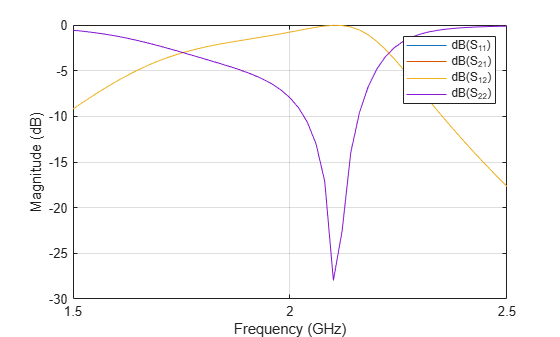### Shifting Resonators and Feed Lines

You can also shift the resonators and the feeds using the `ResonatorOffset` and `FeedOffset` properties of `filterHairpin.`

Change the `ResonatorOffset `and `FeedOffset `and visualize it.

```filter.ResonatorOffset = [0e-3 3e-3 5e-3]; filter.FeedOffset(2) = filter.FeedOffset(2) + 5e-3; figure; show(filter); view(-37,37);```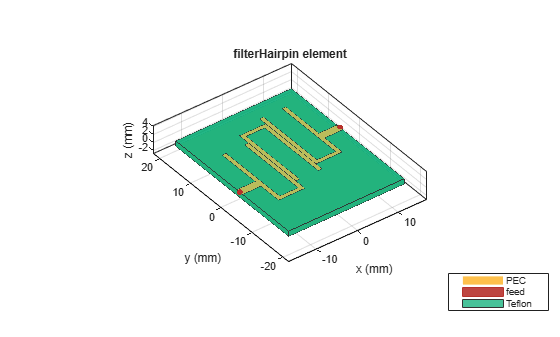### Comparing Results with the Paper

Change the dimensions of the filter as given in . Change the `spacing` on the `filterHairpin `and set the spacing to 0.4 mm and set the length of the U-Shaped resonator to 2 mm.

```filter.ResonatorOffset = [0e-3 0e-3 0e-3]; filter.Resonator(1).Length(1) = 20.4e-3; filter.Resonator(1).Length(2) = 2e-3; filter.Resonator(1).Length(3) = 20.4e-3; filter.Spacing = [0.4e-3 0.4e-3]; filter.PortLineWidth = 1.85e-3; filter.FeedOffset = [-4.565e-3 -4.565e-3]; figure; show(filter); view(-37,29);```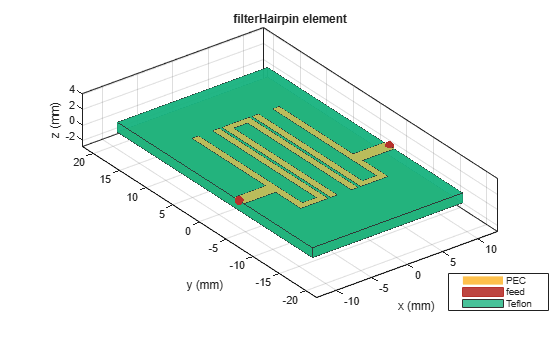Use the `sparameters` function to calculate the s-parameters for the hairpin filter and plot it using `rfplot` function.

```spar = sparameters(filter,f); figure; rfplot(spar); title('S-Paramters for the Hairpin Filter');```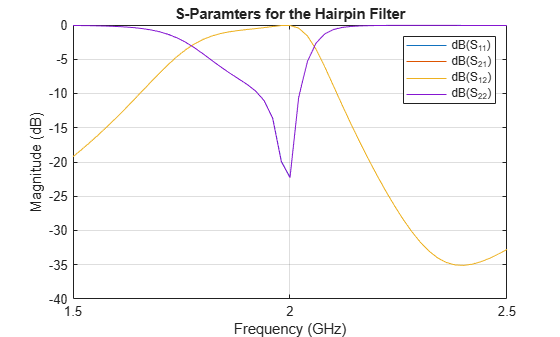Plot the absolute values of S21 over the frequency range using `rfplot` function.

```figure; rfplot(spar,2,1,'abs'); title('Magnitude - S21');```### Coupled-Input Hairpin Filter

Use the `filterHairpin` object to create the hairpin filter. Change the `FeedType` property to `Coupled` to create the hairpin filter with a coupled Input.

```filterC = filterHairpin; filterC.FeedType = 'Coupled'; filterC.FeedOffset = [0 0]; figure; show(filterC); view(-37,35);```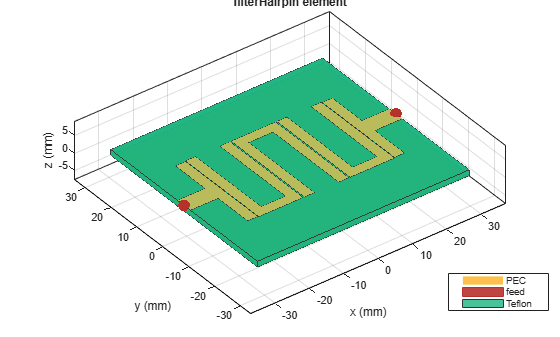Use the `sparameters` function to calculate the s-parameters for the hairpin filter and plot it using `rfplot` function. The frequency range for the simulation is taken as 1.5 GHz to 2.5 GHz with 301 points. As this below simulation takes more time to run, load the sparameters from the s2p file and then plot the S-Parameters.

```sparC = sparameters('sparams.s2p'); figure; rfplot(sparC);```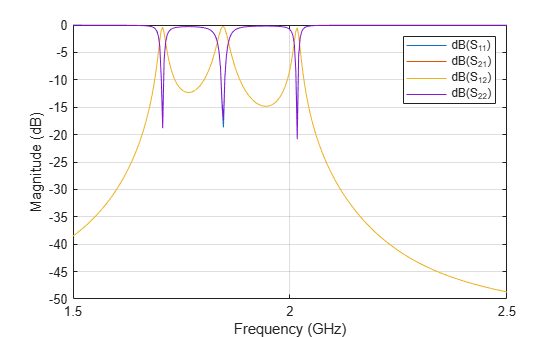The result shows narrow band resonances at 1.7 GHz, 1.85 GHz, and 2 GHz. For this type of Input, the `ResonatorOffset` and `FeedOffset` can be used to create different types of Hairpin Filters.

### References

1. Nikunj Parikh , Pragya Katare , Ketan Kathal , Nandini Patel , Gaurav Chaitanya, Design and Analysis of Hairpin Micro-Strip Line Band Pass Filter.